### Home > INT2 > Chapter Ch12 > Lesson 12.2.4 > Problem12-144

12-144.
1. 12-144.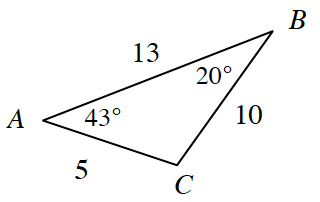For each triangle below, decide if it is similar to the triangle at right. If it is similar, justify your conclusion and complete the similarity statement ∆ABC ~∆_____. If the triangle is not similar, explain how you know. Assume that the diagrams are not drawn to scale. Homework Help ✎

2.  a.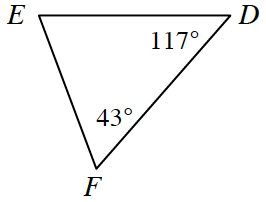b.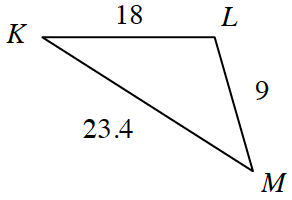c.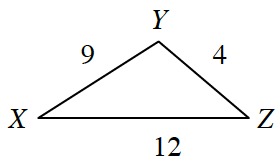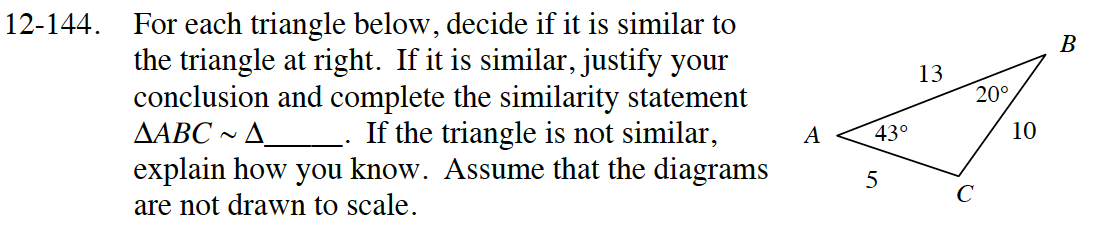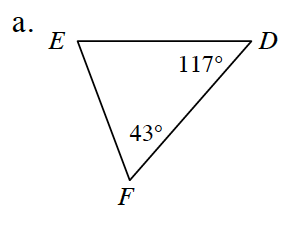Solve for the third angle of each triangle. What do you notice?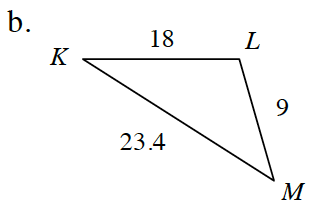Scale factors may not necessarily be whole numbers.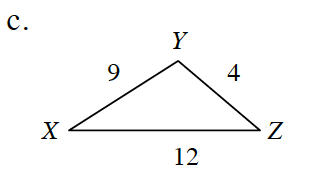How is this triangle different from that of part (b)?

Not similar. Why?